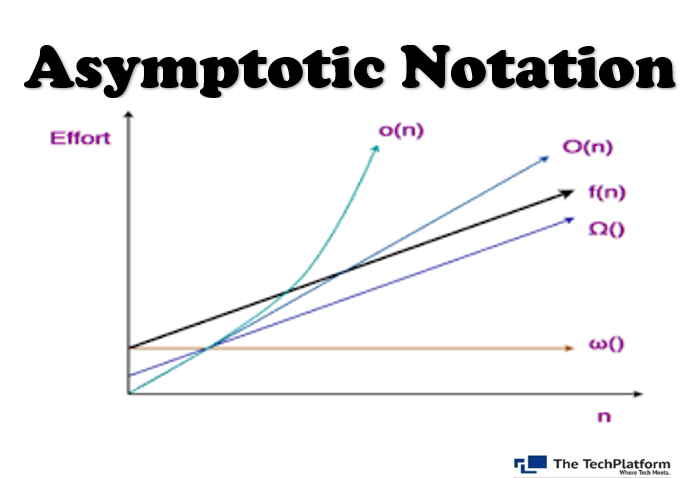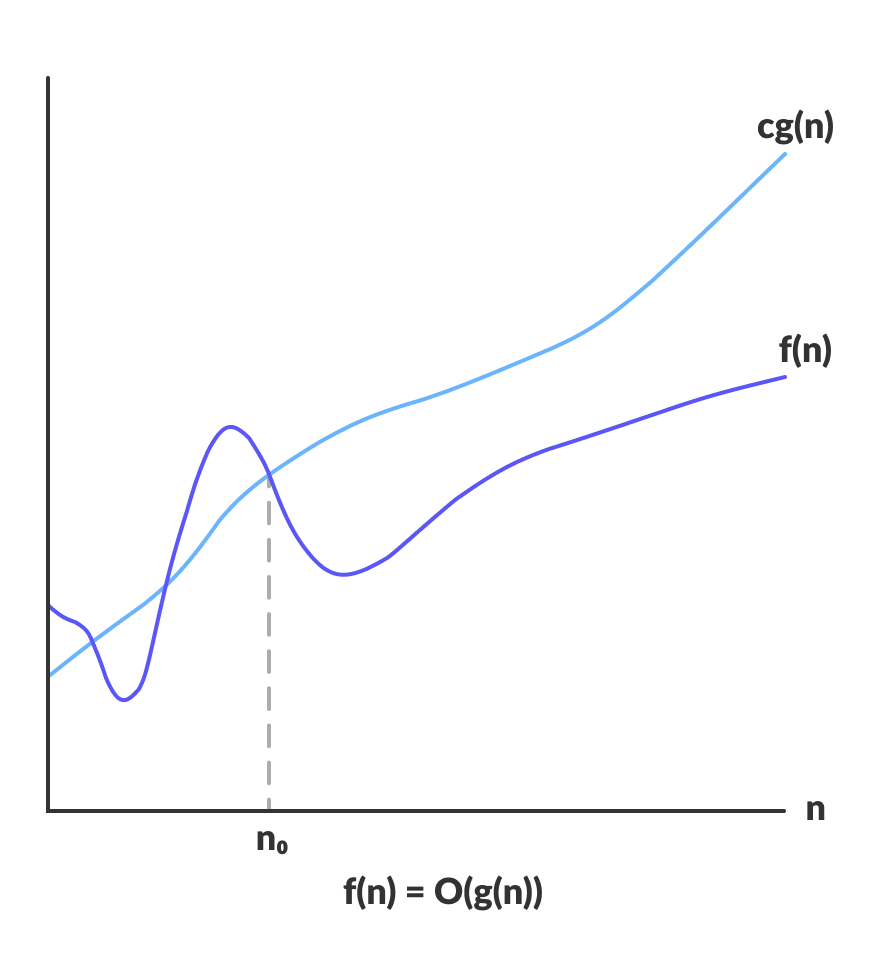top of page
Search

# Asymptotic NotationAsymptotic Notation is used to describe the running time of an algorithm - how much time an algorithm takes with a given input, n. There are three different notations: big O, big Theta (Θ), and big Omega (Ω). big-Θ is used when the running time is the same for all cases, big-O for the worst case running time, and big-Ω for the best case running time.

Asymptotic notations are the mathematical notations used to describe the running time of an algorithm when the input tends towards a particular value or a limiting value.

Usually, the time required by an algorithm falls under three types −

• Best Case − Minimum time required for program execution.

• Average Case − Average time required for program execution.

• Worst Case − Maximum time required for program execution.

There are mainly three asymptotic notations:

• Big-O notation

• Omega notation

• Theta notation

## Big-O Notation (O-notation)

Big-O notation represents the upper bound of the running time of an algorithm. Thus, it gives the worst-case complexity of an algorithm.

Big-O gives the upper bound of a function

```O(g(n)) = { f(n): there exist positive constants c and n0
such that 0 ≤ f(n) ≤ cg(n) for all n ≥ n0 }```

The above expression can be described as a function f(n) belongs to the set O(g(n)) if there exists a positive constant c such that it lies between 0 and cg(n), for sufficiently large n.For any value of n, the running time of an algorithm does not cross the time provided by O(g(n)).

Since it gives the worst-case running time of an algorithm, it is widely used to analyze an algorithm as we are always interested in the worst-case scenario.

## Omega Notation (Ω-notation)

Omega notation represents the lower bound of the running time of an algorithm. Thus, it provides the best case complexity of an algorithm.Omega gives the lower bound of a function

```Ω(g(n)) = { f(n): there exist positive constants c and n0
such that 0 ≤ cg(n) ≤ f(n) for all n ≥ n0 }```

The above expression can be described as a function f(n) belongs to the set Ω(g(n)) if there exists a positive constant c such that it lies above cg(n), for sufficiently large n.

For any value of n, the minimum time required by the algorithm is given by Omega Ω(g(n)).

## Theta Notation (Θ-notation)

Theta notation encloses the function from above and below. Since it represents the upper and the lower bound of the running time of an algorithm, it is used for analyzing the average-case complexity of an algorithm.Theta bounds the function within constants factors

For a function g(n), Θ(g(n)) is given by the relation:

```Θ(g(n)) = { f(n): there exist positive constants c1, c2 and n0
such that 0 ≤ c1g(n) ≤ f(n) ≤ c2g(n) for all n ≥ n0 }```

The above expression can be described as a function f(n) belongs to the set Θ(g(n)) if there exist positive constants c1 and c2 such that it can be sandwiched between c1g(n) and c2g(n), for sufficiently large n.

If a function f(n) lies anywhere in between c1g(n) and c2g(n) for all n ≥ n0, then f(n) is said to be asymptotically tight bound.

## Common Asymptotic Notations

Following is a list of some common asymptotic notations −1. Asymptotic notations help us represent the upper and lower bound of execution time in the form of mathematical equations.

2. It is not feasible to manually measure the performance of the algorithm as it changes with the input size. In such a case, asymptotic notations help us give a general insight into execution time for an algorithm.

3. Asymptotic analysis is so far the most efficient and the best way to analyse algorithms against the actual running time.

4. Asymptotic analysis helps us in performing our task with fewer efforts.

The Tech Platform